# Short Notes: Electric Charges and Fields – Part 1# Types of Charges

When an ebonite rod is rubbed with wool, the ebonite rod acquires negative charge. When a glass rod is rubbed with silk, the glass rod acquires positive charge.

In fact when two substances are rubbed together, one acquires negative charge and the other acquires equal amount of positive charge.

Thus, there are two types of charges:

(i) Positive charge (ii) Negative charge

The names positive and negative charges were given arbitrarily.

# Electronic Theory of ElectrificationEvery matter is composed of tiny particles called the atoms. According to Rutherford atomic model, an atom consists of a tiny nucleus at its centre containing whole positive charge called proton and almost whole mass of the atom. The nucleus is surrounded by negatively charged electrons. These electrons revolve around the nucleus in circular/elliptical orbit. The electrons far off from the nucleus are weakly bound and they can easily be ditached. Only these negatively charged electrons are responsible for electrification of bodies. For example, when glass rod is rubbed with silk, the electrons of glass rod are transferred to silk; so glass rod loses electrons and acquires positive charge; while silk gains electrons and acquires equal amount of negative charge. The S.I. unit of charge is coulomb abbreviated as C.ss

The nucleus consists of positively charged protons and neutral neutrons; both protons and neutrons are collectively called the nucleons.

Hence, The constituent particles of atom are:

 Charge Mass Discoverer (i) Proton +1.6 × 10-19C (or + e) 1.673 × 10-27 kg Goldstein (ii) Neutron Zero 1.675 × 10-27 kg Chadwick (iii) Electron +1.6 × 10-19C (or + e) 9.1 × 10-31 kg Thomson

Note: The name electron is given by Sir J. Stoney. Concept of electron started with Millikan’s experiment

# Properties of Charges

(ii) Charge is conserved

(iii) Charge is quantised

(iv) Charge is unaffected by motion

(v) A charged body attracts light uncharged bodies

(vi) Similar charges repel each other but opposite charges attract.

# Conservation of Charge

It states that the charge of an isolated system remains constant. This means that the charge can neither be created nor destroyed; but it may simply be transferred from one body to the other. For example, when two neutral bodies (initial charge zero) are rubbed together, one acquires positive charge and the other equal negative charge. The net charge of both the bodies is zero, as it was before rubbing. Hence charge is conserved.

# Quantisation of Charge

The net charge on a body is always an integral multiple of the fundamental charge  (e = 1.6 × 10-19) C i.e.,

q = ne

where n is any integer, positive or negative.

The principle of quantisation of charge is obvious because charge on a body is produced due to transfer of electrons and electron is indivisible.

Quark – Latest developed (discovered) fundamental sub-particle.

The smallest possible charge is of ‘Quark’but experimentally it is not confirmed.

# Coulomb’s Law

Experimental observations reveal that similar charges repel each other and opposite charges attract.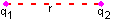Coulomb’s law states that the force of repulsion or attraction between two point charges is directly proportional to the product of the charges and inversely proportional to the square of distance between them. The direction of the force is along the line joining the two charges. If q1 are two point charges at a separation r, then q2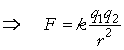Thus 1 coulomb charge is the charge which when placed at a distance of 1 metre from an equal and similar charge in vacuum (or air) repels it with a force ofN.

# Dielectric Constant

The dielectric constant (K) of a medium is defined as the ratio of permittivity of medium to the permittivity of free space, i.e.,

ε0

When space between two charges is filled with a medium of absolute permittivity

ε0 = 8.85 × 10-12 colomb2⁄ newton meter2(in SI units) then Coulomb’s force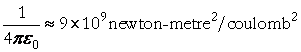Therefore, the force in medium is reduced to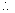times of that in air.

# Coulomb`s law in vector form

Force is a vector quantity, therefore, its direction must be specified. If q1 are two charges at separation r, then the magnitude of force is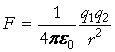and its direction is along the line joining ε.

q1= q= 1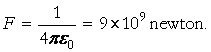9  × 109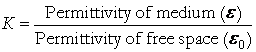That is Newton’s third law holds also for electric forces.